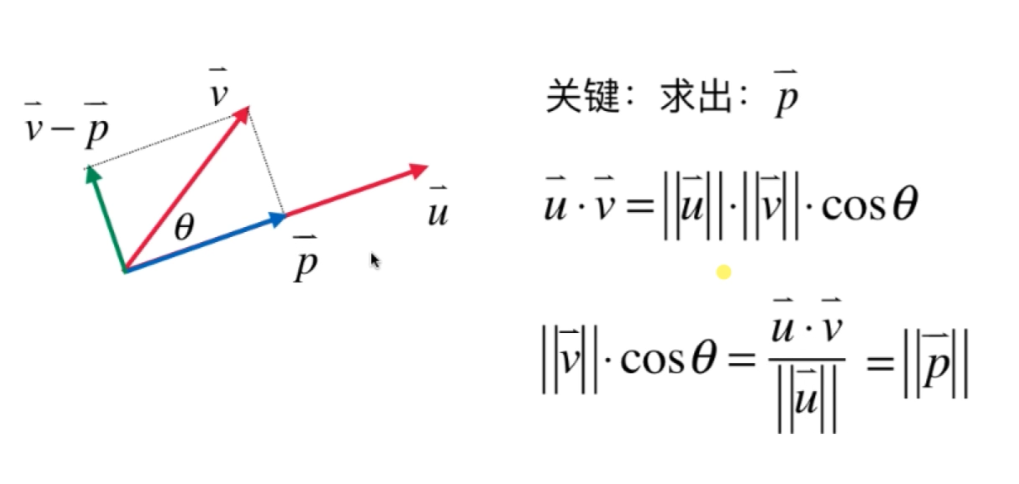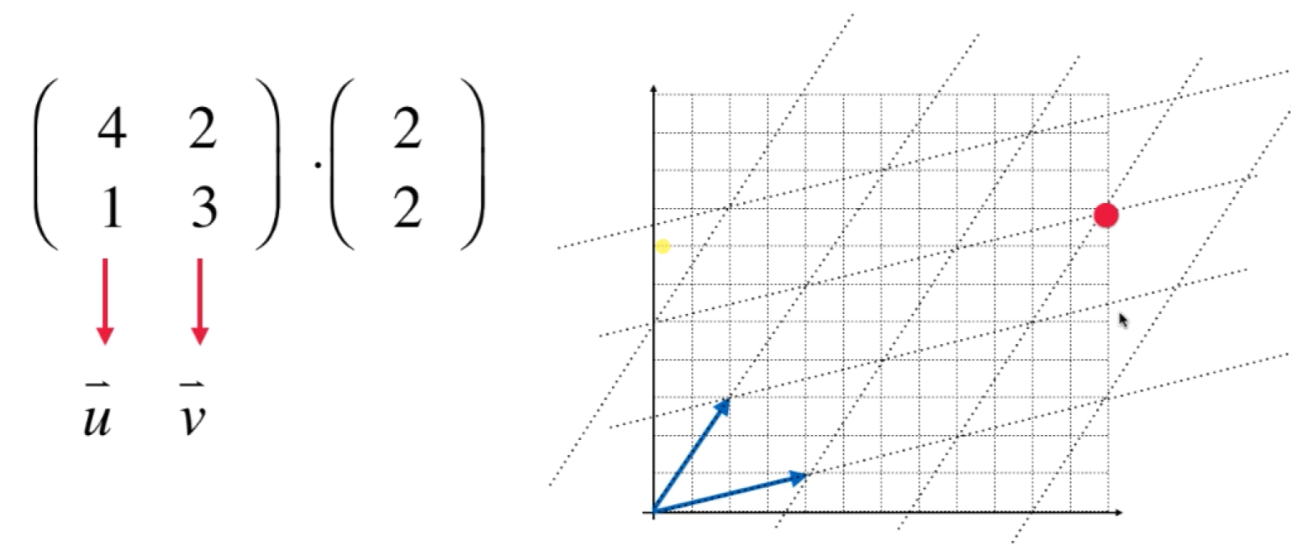### 正交基和标准正交基¶

n维空间中，任意n个线性无关的向量，一定是这个n维空间的基。
n个非零正交向量一定是n维空间的基。

### 一维投影¶因为是向量运算因此$\vec{u}$是不能做约分的

### 高维投影¶此为 Gram-Schmidt 过程（注意，算法的输入是一组基，即线性无关的）

### 标准正交矩阵¶• $Q^T \cdot Q = I$
• $Q$的各列线性无关
• $Q$可逆
• $Q^T$是$Q$的左逆
• $Q^T$是$Q$的右逆
• $Q^T$是$Q$的逆
• $Q^{-1}=Q^T$

### 矩阵的QR分解¶

$A=QR \longrightarrow R=Q^{-1}A \longrightarrow R=Q^T A$